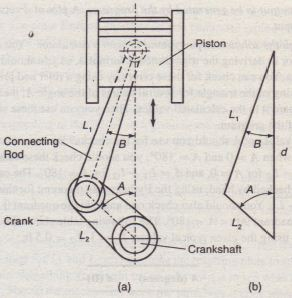Piston Motion

Figure 1.7-2a shows a piston, connecting rod, and crank for an internal combustion engine. When combustion occurs, it pushes the piston down. This motion causes the connecting rod to turn the crank, which causes the crankshaft to rotate. We want to develop a MATLAB program to compute and plot the distance d traveled by the piston as a function of the angle A, for given values of the lengths LI and L2• Such a plot would help the engineers designing the engine to select appropriate values for the lengths LI and L2.

We are told that typical values for these lengths are L 1 = If t and L2 = 0.5 ft. Because the mechanism’s motion is symmetrical about A =0, we need consider only angles in the range 0 ≤ A ≤ 1800 Figure 1.7-2b shows the geometry of the motion. From this figure we can use trigonometry to write the following expression-for d:Figure 1.7-2 A piston, connecting rod, and crank for an internal combustion engine.

Thus to compute d given the lengths L 1 and L2 and the angle A. we must first determine the angle B. We can do so using the law of sines. as follows:Equations (1.”-2) and (1.7-3) form the basis of our calculations. Develop and test a  MATLAB program to plot d versus A.

Solution

Here are the steps in the solution. following those listed in Table 1.7-2.

1. State the problem concisely. Use equations (1.7-2) and (1.7-3) to compute d; use
enough values of A in the range 0 ≤ A ::: 1800 to generate an adequate (smooth) plot.
2. Specify the input data to be used by the program. The lengths LI and L2 and the angle A are given.
3. Specify the output to be generated by the program. A plot of d versus A is the required output.
4. Work through the solution steps by hand or with a calculator: You could have made an error in deriving the trigonometric formulas, so you should check them for several cases. You can check for these errors by using a ruler and protractor to make a scale drawing of the triangle for several values of the .angle A, measure the length d, and compare it to the calculated values. Then you can use these results to check the output of the program.
Which values of A should you use for the checks? Because the triangle “collapses” when A = 0 and A = 180°; you should check these cases. The results are d = £1 -!-2 for A =·0, and d = LI +1.2 for A = 180°.The case A = 90° is also easily checked by hand, using the Pythagorean theorem; for this case d = v
5. Li – L.You should also check one angle in the quadrant 0 < A < 90° and one in the quadrant 90° < A < 180’1.The following table shows the results of these calculations using the given typical values: L 1 = 1, L2 = 0.5 ft.6. Write and run the program. The following ~AB session uses the values
L1= 1, L2 = 0.5 ft.Note the use of the underscore C) in variable names to make the names more meaningful. The variable A_d represents the angle A in degrees. Line 4 creates an array of numbers 0, 0.5, I, 1.5, … , 180. Line 5 converts these degree values to radians and assigns the values to the variable A_r. This conversion is necessary because MATLAB trigonometric functions use radians, not degrees. (A common oversight is to use degrees.) MATLAB provides the built-in constant pi to use for It . Line 6 uses the inverse sine function asin.
The plot command requires the label and grid commands to be on the same line, separated by commas. The line-continuation operator, called an ellipsis. consists of three periods. This operator enables you to continue typing the line after you press Enter. Otherwise, if you continued typing without using the ellipsis, you would not see the entire line on the screen. Note that the prompt is not visible when you press Enter after the ellipsis.
The gr id command puts grid lines on the plot so that you can read values from the plot more easi!y. The resulting plot appears in Figure 1.7-3.
7. Check the output of the program with your hand solution. Read the values from the plot corresponding to the values of A given in the preceding table. You can use the input function to read values from the plot. The values should agree with each
other and they do.
8. Run the program and perform a “reality check” on the output. You might suspect an error if the 1’lot showed abrupt changes or discontinuities. However, the plot is smooth and shows that d behaves as expected. It decreases smoothly from its maximum ! it A =·0 to its minimum at A = 1800.
9. Test the program for a range of reasonable input values. Test the program using various values for L I and L2 and examine the resulting plots to see whether they are reasonable. Something you might try on your own is to see what happens. Should the mechanism work the same way it does when What does your intuition tell you to expect from the mechanism? What does the program predict?